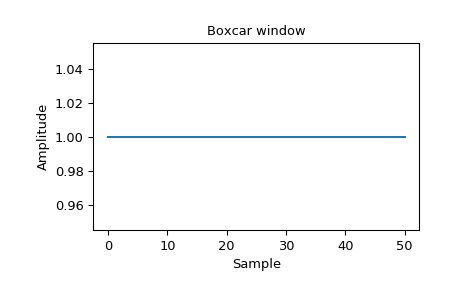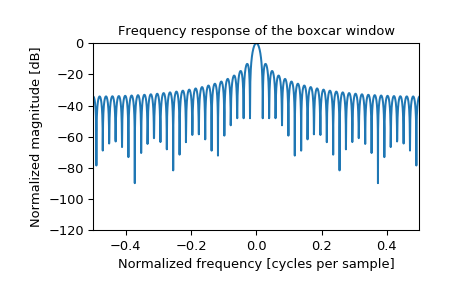# scipy.signal.boxcar¶

scipy.signal.boxcar(M, sym=True)[source]

Return a boxcar or rectangular window.

Also known as a rectangular window or Dirichlet window, this is equivalent to no window at all.

Parameters: M : int Number of points in the output window. If zero or less, an empty array is returned. sym : bool, optional Whether the window is symmetric. (Has no effect for boxcar.) w : ndarray The window, with the maximum value normalized to 1.

Examples

Plot the window and its frequency response:

>>> from scipy import signal
>>> from scipy.fftpack import fft, fftshift
>>> import matplotlib.pyplot as plt

>>> window = signal.boxcar(51)
>>> plt.plot(window)
>>> plt.title("Boxcar window")
>>> plt.ylabel("Amplitude")
>>> plt.xlabel("Sample")

>>> plt.figure()
>>> A = fft(window, 2048) / (len(window)/2.0)
>>> freq = np.linspace(-0.5, 0.5, len(A))
>>> response = 20 * np.log10(np.abs(fftshift(A / abs(A).max())))
>>> plt.plot(freq, response)
>>> plt.axis([-0.5, 0.5, -120, 0])
>>> plt.title("Frequency response of the boxcar window")
>>> plt.ylabel("Normalized magnitude [dB]")
>>> plt.xlabel("Normalized frequency [cycles per sample]")#### Previous topic

scipy.signal.bohman

#### Next topic

scipy.signal.chebwin